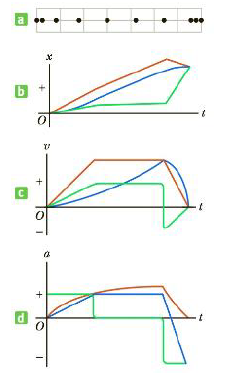Chapter 2.2, Problem 2.5QQ

Chapter
Section
Textbook Problem

Figure 2.14a is a diagram of a multiflash image of an air puck moving to the right on a horizontal surface. The images sketched are separated by equal time intervals, and the first and last images show the puck al rest. (a) In Figure 2.14b, which color graph best shorn the puck’s position as a function of time? (b) In Figure 2.14c, which color graph best shows the puck’s velocity as a function of time? (c) In Figure 2.l4d, which color graph best shows the puck's acceleration as a function of time?Figure 2.14 (Quick Quiz 2.5) Choose the correct graphs.

(a)

To determine

The graph shows the puck’s position as a function of time.

Explanation

Given Info: The distance of the puck is represented in Figure 2.14 and the position versus time graph is represented in Figure 2.14 b.

The distance covered is three times than the initial distance travelled in the first interval. In the following four intervals, the rate is steady...

(b)

To determine

The graph shows the puck’s velocity as a function of time.

(c)

To determine

The graph shows the puck’s acceleration as a function of time.

Still sussing out bartleby?

Check out a sample textbook solution.

See a sample solution

The Solution to Your Study Problems

Bartleby provides explanations to thousands of textbook problems written by our experts, many with advanced degrees!

Get Started